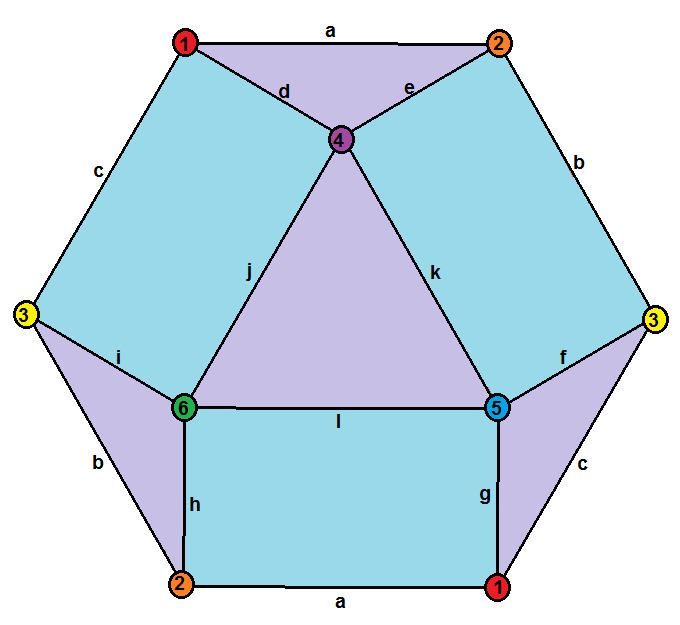# Hemi cuboctahedron

Updated on
 Edges  12Vertex configuration  3.4.3.4 Vertices  6Schläfli symbol  r{3,4}/2 or r{3,4}3Type  abstract polyhedron globally projective polyhedronFaces  7: 4 triangles 3 squares

A hemi-cuboctahedron is an abstract polyhedron, containing half the faces of a semiregular cuboctahedron.

## Contents

It has 4 triangular faces and 3 square faces, 12 edges, and 6 vertices. It can be seen as a rectified hemi-octahedron or rectified hemi-cube.

It can be realized as a projective polyhedron (a tessellation of the real projective plane by 4 triangles and 3 square), which can be visualized by constructing the projective plane as a hemisphere where opposite points along the boundary are connected.

## Dual

Its dual polyhedron is a rhombic hemi-dodecahedron which has 7 vertices (1-7), 12 edges (a-l), and 6 rhombic faces (A-F).

## Related polyhedra

It has a real presentation as a uniform star polyhedron, the tetrahemihexahedron.

Topics